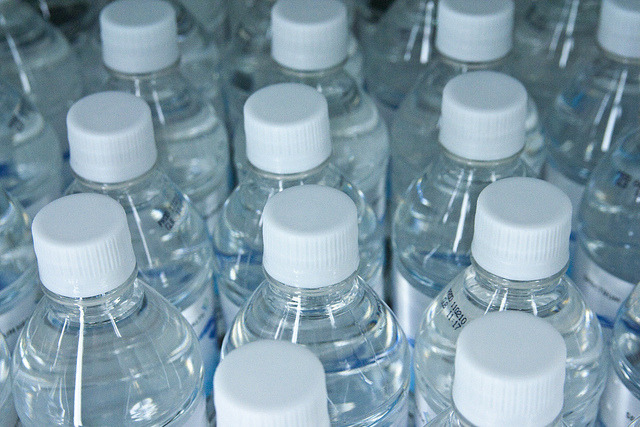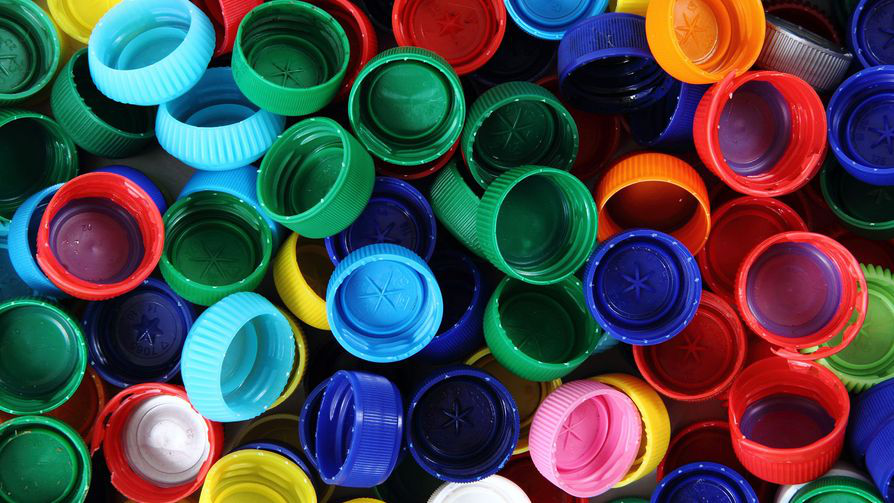Subject:
Statistics and Probability
Material Type:
Lesson Plan
Level:
Middle School
7
Provider:
Pearson
Tags:
• Experimental Probability
Language:
English
Media Formats:
Text/HTML

# The Law Of Large Numbers## Overview

Students will extend their understanding of probability by continuing to conduct experiments with outcomes that do not have a theoretical probability. They will make predictions on the number of outcomes from a series of trials, and compare their predictions with the experimental probability calculated from an experiment.

# Key Concepts

• Students continue to investigate the Law of Large Numbers.

# Goals and Learning Objectives

• Deepen understanding of experimental probability.
• Use proportions to predict results for a number of trials and to calculate experimental probability.
• Understand that some events do not have theoretical probability.
• Understand that there are often many factors involved in determining probability (e.g., human error, randomness).

# Lesson Guide

Have students examine the bottle caps. Students will briefly discuss things they notice.

# Mathematics

Students should see that the outcomes are not equally likely, mostly because the weight is not evenly distributed.

Explain that some situations do not have theoretical probability, particularly if the outcomes are not equally likely. In the case of dropping a bottle cap, there is no way to tell what the theoretical probability of each outcome is, even though we may have a fairly good idea of how things might turn out. The probability is established as more and more trials are performed. A good example is to look at how insurance companies determine rates by looking at statistics and determining probability over time. Another example students have seen is predicting weather (e.g., there is a 40% chance of rain tomorrow).

SWD: Predicting outcomes using theoretical probability can be challenging for some students with disabilities. Review and reinforce this skill with students prior to having them start the tasks in which they will be expected to predict outcomes for various situations.

# Bottle Cap Landings

Suppose you toss a plastic bottle cap in the air.

• What are the possible ways it could land?
• Do you think these outcomes are equally likely?
• Discuss the possible outcomes with your classmates.# Lesson Guide

Discuss the Math Mission. Students will find the probabilities of events that do not have equally likely outcomes.

## Opening

Find the probabilities of events that do not have equally likely outcomes.

# Lesson Guide

Students will work individually to collect and enter their data, although they are encouraged to talk to their neighbors and compare results.

To drop the bottle cap, students should flip the cap about a foot above their desk. Discuss the importance of everyone following the same procedure in order to get random, unbiased results.

Once each student has conducted their experiment, they should record their results in the Bottle Caps interactive. Then, collect your class results and make a line plot for each outcome.

Students then use these results to calculate the experimental probability of each item based on the class results.

As students work, look for examples of different ways that students found the probability based on 30 trials, and predicted results for 900 trials.

• Did any students use proportions, decimals, or percentages?
• Did any students multiply the results for 30 by 30 to predict the number of outcomes for 900 trials?

# Interventions

Student has a prediction of the results of 900 trials, but does not provide an explanation.

• Why did you approach the problem the way you did?
• Explain your strategy for solving the problem.
• How did you know that multiplying would work?

• Answers will vary. Answers depend on the results of students' experiments.
• Answers will vary. Answers depend on the results of students' experiments and initial guesses.
• Answers will vary. Answers depend on the results of students' experiments.
• Answers will vary. Answers depend on the results of all students' experiments.

# Bottle Cap Experiments

Suppose you drop a plastic bottle cap 30 times.

• Predict how many times it will land open side up, open side down, or on its side. Record your guesses in the Bottle Caps interactive table.
• Drop a bottle cap 30 times and record your results.

• Use your results from the 30 trials to calculate the experimental probability of each outcome.
• Based on these probabilities, predict the results for each outcome if you were to conduct 900 trials.

Your teacher will share the class results for the 30 trials.

• Calculate the experimental probability of each outcome based on the class results.

INTERACTIVE: Bottle Caps

# Lesson Guide

Students will work with a partner to create a spinner to simulate the bottle cap experiment.

Pairs should combine their results so that they are creating the spinner based on data from 60 drops.

Look at ways that students create the spinner. Some may look at the proportion of each outcome compared to the circle, while others may look at how the probabilities compare to each other. For example, if the bottle cap landed upside down 10 times more frequently than on its side, that section should be 10 times wider.

ELL: Student partner work provides students with opportunities to negotiate meaning, share ideas, and communicate with others during problem solving. This type of language-rich environment encourages students to work together and learn from one another—which is essential for ELLs' understanding.

# Interventions

Student has trouble getting started on making the spinner.

• Did any outcomes occur more than half of the time?
• How would this look on the circle?
• Did any outcomes occur around $\frac{1}{4}$ of the time?
• How would this look on the circle?

• Spinners will vary. Spinners depend on the results of students' experiments.
• Answers will vary. Answers depend on the results of students' experiments.
• Answers will vary. Answers depend on the results of students' experiments.
• Each spinner was based on the results of two students, each of which most likely had different results because of the small number of trials. The spinners are probably not an accurate reflection of the established probability.

# Create a Spinner

• Work with a partner to create a spinner that simulates the probabilities for dropping the bottle cap. Use the Blank Spinner interactive.
• Spin the spinner 30 times and save your results.
• Calculate the experimental probability of each event based on your results.
• How do your experimental probabilities based on the spinner compare to the experimental probabilities based on the class results from dropping the cap?

INTERACTIVE: Blank Spinner

# Lesson Guide

Presentations will vary. Presentations should note differences between spinner results and bottle cap results, and differences in results of experiments with a greater number of experiments.

# Preparing for Ways of Thinking

Look for examples that will allow you to formalize definitions during Ways of Thinking:

• Theoretical probability: the ratio of favorable outcomes to the total number of possible outcomes, often just called probability
• Experimental probability: the ratio of favorable outcomes to the total number of trials
• Outcome: a single possible result from an experiment
• Event: a set of possible outcomes from a particular experiment
• Experiment: in probability, a controlled, repeated process (such as tossing a coin)
• Trial: each time the experiment is performed

# Mathematical Practices

Mathematical Practice 1: Make sense of problems and persevere in solving them.

• Students continue to reason about and solve probability problems, deepening their intuition.

Mathematical Practice 2: Reason abstractly and quantitatively.

• Students must be able to decontextualize their experimental results for a bottle cap, and then apply the calculated probabilities to a different experimental situation.

Mathematical Practice 5: Use appropriate tools strategically.

• In this lesson, students use both concrete models (bottle cap) and digital interactives (spinner) to conduct experiments and better understand the relationship between actual results and theoretical probability.

# Challenge Problem

• Answers will vary. Possible answer: The results for these two experiments will be similar, but not exactly the same.

# Challenge Problem

• In one experiment a bottle cap is dropped 600 times. In another experiment a bottle cap is dropped 6,000 times. How do you think the probabilities from these two experiments will compare? Explain.

# Mathematics

Begin the discussion by showing the line plot for the student guesses. There is probably agreement that the bottle cap won't land on its side too often, and students will probably realize that the bottle cap is more likely to land upside down than right side up. Discuss the range of guesses for each outcome, and that it reinforces the need for experimental results.

Show the line plots for the experiment and ask the following:

• What does the range of results in our guesses tell us?
• What does the range of results tell us about the experimental probability?
• How do these results compare to your predictions?
• What does that tell you about the experimental probability?

• How did you decide what the spinner should look like?
• What was the experimental probability from the 100 spins?
• How did your results for the 100 spins compare to the experimental probability established from the 30 drops of the bottle cap?

Show the class totals from the 100 spins:

• What is the experimental probability for these results?
• How do they compare to your 100 spins and the total class drops?
• How was this data (the total spins for the class) collected?
• Is this data reliable? Why?

As students share, they are beginning to see that a larger number of trials results in a more accurate measure of the experimental probability. Students should begin to have an informal understanding of the Law of Large Numbers.

ELL: When working with ELLs during the Ways of Thinking section:

• Speak clearly and monitor your speech for slang and idiomatic expressions.
• Use intonation, volume, and pauses to help make the meaning clear.
• Rephrase instead of repeating; paraphrase important concepts and directions.

SWD:Some students with disabilities may struggle to explain their mathematical reasoning in words. Provide sentence starters and/or paragraph frames to support students with this task.

# Mathematical Practices

Mathematical Practice 3: Construct viable arguments and critique the reasoning of others.

• When presenting or listening to others present, students must think critically about why results might be different, and whether the arguments for these differences are mathematically sound.

# Ways of Thinking: Make Connections

Take notes about other students’ results and conclusions.

# A Possible Summary

Experimental probability is determined by performing a number of trials; it is the ratio of favorable outcomes to the number of trials. Some experiments don't have theoretical probability, particularly if the outcomes are not equally likely. In this case, the experimental probability is calculated and becomes more accurate with more trials.

# Summary of the Math: Experimental Probability

Write a summary about experimental probability.

## Hint:

• Do you describe what experimental probability is?
• Do you explain how experimental probability is related to theoretical probability?
• Do you explain what happens to the experimental probability as the number of trials increases?

# Lesson Guide

Have each student write a brief reflection before the end of the class. Review the reflections to find out what strategies they have found useful for solving probability problems.

• ELL: Ask some questions to all students, but especially to ELLs, to check for understanding before moving on. In addition, make sure that you provide the summary in written form or as an anchor chart.

# Reflection

Write a reflection about the ideas discussed in class today. Use the sentence starter below if you find them to be helpful.

A strategy that I found useful when solving probability problems is...